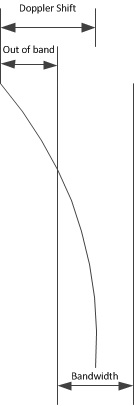# Is it possible to get out of band signal with doppler shift?

Hi!

I have a question re doppler shifting. Currently gr-gpredict-doppler recommends bandwidth configuration: signal bandwidth + max possible doppler shift.

I was wondering how can I predict this shift and avoid the situation shown in the graph below:Changing frequency on hardware device:

• Has non 0 time to apply
• Might be expensive

Sorry for such noob questionNot a noob question at all.

The formula for Doppler shift is relatively simple, see e.g. the Wikipedia article on Doppler effect. We can assume stationary observer:

f = f_0 × (c / (c + v_sat))

Here f_0 is the nominal frequency and v_sat is the velocity of the satellite with respect to the observer (not the orbital velocity). In predict and gpredict this velocity is called “range rate” and you can see that typical values are between 5-10 km/s, depending on the orbit shape and altitude.

If we take 10 km/s we have:

f = f_0 × (299792 km/s / (299792 km/s + 10 km/s)) = 0.999967 × f_0

So, for example, at 436 MHz the Doppler shift will be within ± 20 kHz1 Like

Ah! So satellite should fly with much higher velocity or we should live on Jupiter.

I’m doing some research on current doppler shift calculation algorithms. Found quite interesting issue: https://github.com/brandon-rhodes/pyephem/issues/34 It looks like different algorithms/libraries might calculate doppler differently. Do you think it might worth comparing them? I’m just curiousIt’s always a good idea to compare various algorithms. I know for sure that in Gpredict I use many approximations that are sufficient for practical purposes but not necessarily accurate enough for analytical calculations.

1 Like